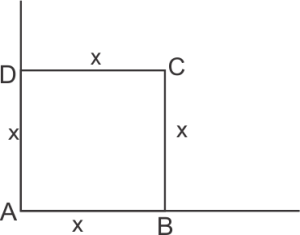# Center of mass Problems for class 11

This article contains Center of mass Problems for class 11. In this article I have given numerical problems, very short answer type questions , short answer type questions and some extra questions.
You can print this article and practice these problems.
You read notes on this chapter before practicing these questions. For them please visit this link
Center of mass
Other assignments(Included with Linear momentum) for this chapter which you can have a look are
Assignment 1
Assignment 2
Assignment 3
Assignment 4


# Center of mass Problems

## Numerical Problems

Question 1 Find the center of mass of a triangular lamina.

Question 2 The masses of 3, 4 and 5 Kg are located at the corners of the equilateral triangle of side 1 m. Locate the center of mass of the system.

Question 3 The particles of masses 100 gm and 300 gm at a given time have positions $2\hat i +5 \hat j + 13 \hat k$ and $-6\hat i +4 \hat j -2 \hat k$ m respectively and velocities $10\hat i -7 \hat j -3 \hat k$ and $7\hat i -9 \hat j + 6 \hat k$ m/s respectively. Determine the instantaneous position and velocity of CM.

Question 4 Show that the center of mass of a uniform rod of mass $M$ and length $L$ lies at the middle point of the rod.

Question 5 Determine the center of mass of a hemisphere of radius $R$.

Question 6 A circular plate of uniform thickness has a diameter 56 cm. A circular portion of 42 cm is removed from one edge of the plate. Find the CM of the remaining portion.

Question 7 The identical spheres A, B and C each of radius R are placed touching each other on a horizontal table. Where is the center of mass of the system located relative to center of mass of the sphere A?

Question 8 Two blocks of mass 2 Kg and 5 Kg are placed on a frictionless table and connected by a spring. On giving a jerk, the heavier block starts moving towards the other block with velocity 9.8 m/s. Find the velocity of the center of mass.

Question 9 A thin rod of length $l$ and mass $m$ has a disk which is attached to one of its ends such that rod and disk are coplanar. Find CM from the center of disk, if mass of the disk is M and radius is $R$.

Question 10 Four particles of masses 2m, m , 4m and 3m are placed at the corners A, B, C and D of a square of each side x as shown below in the figure.Find the position of the center of mass of the system.

## Very Short answer type questions

Question 1 Where does the center of mass of a two particle system lie , if one particle is more massive then another.

Question 2 Is center of mass a reality?

Question 3 Where does center of mass of a rectangle lie?

Question 4 Should center of mass of a body necessarily lie inside the body?

Question 5 What will be nature of motion of center of mass of an isolated system?

Question 6 How is the center of mass depends on the relative distance between the particles?

Question 7 Write two factors on which center of mass of a body does not depend.

Question 8 Give the location of a center of mass of a ring? does it it lie inside the body?

Question 9 Define motion of center of mass of a fire cracker that explode in air before and after exposition?

## Short Answer type questions

Question 1 A body A of mass M while falling vertically downwards under the gravity breaks into two parts; a body B of mass M/3 and a body C of mass 2M/3. How does the center of mass of bodies B and C taken together shift compared to that of body A?

Question 2 Prove that the center of mass of two particles divides the line joining the particles in the inverse ratio of their masses.

Question 3 Distinguish between the center of gravity of a body and its center of mass.

Question 4 Two identical particles move towards each other with velocity $2v$ and $v$ respectively. What is the velocity of the center of mass?

Question 5 What is the significance of defining center of mass of a system?

## Extra Questions

Question 1 Obtain an expression for the position vector of the center of mass of a system containing two particles.

Question 2 Discuss the motion of center of mass in the following systems

(a) Earth moon system revolving around the sun

(b) Diatomic molecule

Question 3 What do you mean by center of mass of a system. Prove that center of mass of a system moves with constant velocity in the absence of external force on the system.

Answers to selected Center of mass Problems

Question 1

CM lies on the point of concurrence of the medians i.e., on the centroid G of the triangle.

Question 2

The coordinates of CM are (0.54 m, 0.36 m)

Question 3

${{\vec{R}}_{CM}}=\frac{-16\hat{i}+17\hat{j}+7\hat{k}}{4}m$

${{\vec{v}}_{CM}}=\frac{31\hat{i}-34\hat{j}+15\hat{k}}{4}m{{s}^{-1}}$

Question 6

9 cm

Question 7

$\frac{1}{3}(\vec{AB}+\vec{AC})$

Question 8

7 ms-1

Question 9

${{X}_{CM}}=\frac{m(2r+l)}{2(M+m)}$

Question 10

(0.5x , 0.7x)

Some other resources you can look for reference are
https://en.wikipedia.org/wiki/Center_of_mass
http://hyperphysics.phy-astr.gsu.edu/hbase/cm.html
Hope you like this Center of mass Problems for practice and these help you in your exams. If you like this article please share it among your friends.

0 Followers

Most reacted comment
4 Comment authors

This site uses Akismet to reduce spam. Learn how your comment data is processed.

Subscribe
Notify of
Unknown